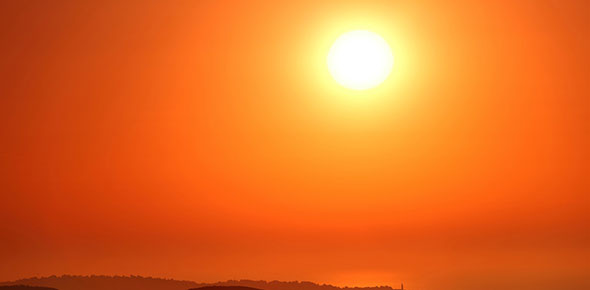# Sun Quiz: Physical Properties & The 8 Layers

17 Questions | Total Attempts: 4499SettingsThis quiz will determine the amount of knowledge acquired from this VFT exploring the Sun.

• 1.
How does the Sun rotate?
• A.

Differentially

• B.

Clockwise

• C.

Counter-clockwise

• D.

Faster at the poles, slower at the equator

• 2.
The light we see from the Sun comes from which layer?
• A.

Ionosphere

• B.

Troposphere

• C.

Photosphere

• D.

Corona

• 3.
The temperature of the layer of gas that produces the visible light of the Sun is:
• A.

15 million K

• B.

5,800 K

• C.

90,000 K

• D.

12, 540 K

• 4.
A loop of gas following the magnetic field lines between sunspots' poles is:
• A.

A coronal hole

• B.

A solar flare

• C.

A ray

• D.

A prominence

• 5.
How many planet Earths could fit inside the Sun?
• A.

1,000

• B.

Close to a billion

• C.

A little over a million

• D.

2,000

• 6.
The density of the Sun is most similar to which object?
• A.

Jupiter

• B.

The Earth

• C.

Haleys Comet

• D.

Mercury

• 7.
Energy generated by nuclear fusion occurs where?
• A.

• B.

Core

• C.

Chromosphere

• D.

Corona

• 8.
Which layer is energy transported by electromagnetic radiation?
• A.

Core

• B.

• C.

Photosphere

• D.

Corona

• 9.
What happens in the photosphere?
• A.

• B.

Nuclear fusion

• C.

Nuclear fission

• D.

Energy carried by convection

• 10.
Cool lower atmosphere:
• A.

Solarsphere

• B.

Borosphere

• C.

Crasmphere

• D.

Chromosphere

• 11.
What layer(s) can solar material escape into space and flow outward through the solar system?
• A.

• B.

Solar wind

• C.

• D.

The core & the chromosphere

• 12.
In the radiation zone of the Sun the temperature is a little cooler than the core and as a result some atoms are able to remain intact. In this manner the energy that is generated in the core is passed from atom to atom through the:
• A.

Transition zone

• B.

Chromosphere

• C.

Core

• D.

• 13.
The exterior of the Sun is comprised of the _______ and the______, both of which can be studied using an array of telescopes and radiation detectors.
• A.

Solar contant and the surface

• B.

Solar oscillation and solar constant

• C.

Stars and the milkyway

• D.

Surface and the atmosphere

• 14.
The continuous rising and falling of hot and cool bubbles produces a pattern on the surface of the Sun that is referred to as:
• A.

Flares

• B.

Transuslation

• C.

Solar oscillation

• D.

Granulation

• 15.
Energy is transported through the photosphere by:
• A.

• B.

Xrays

• C.

• D.

UV rays

• 16.
It takes light from the Sun how long to reach Earth:
• A.

24 hours

• B.

1 minute

• C.

10 seconds

• D.

Just over 8 minutes

• 17.
Most of the light that we receive from the Sun on earth is energy that was released by atoms in the:
• A.

Photosphere

• B.

Platasphere

• C.

Chromosephere

• D.

Corona

Related TopicsBack to top